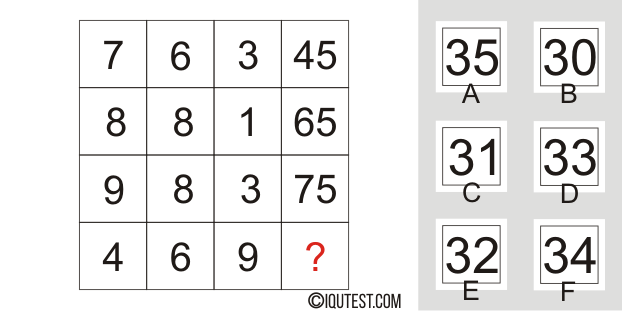# IQ Test Series #6

This is a series #6 with a total of 30 questions of fun and challenging I.Q Test with variable type of questions like basic numeracy, numbers, math, spatial ability and logical.

You can practice your lateral thinking, problem solving and reasoning skills. Improve your performance on standard IQ Test questions, brainpower and greater mental task with other our series of IQ Tests.

Example Question:
What should replace the question mark?Explanation: (7 x 6) + 3 = 45 | (8 x 8) + 1 = 65 | (9 x 8) + 3 = 75

(4 x 6) + 9 = 33

IQ Test Series #6
• 30 questions
• Mathematical, Logical, Spatial
• Time Limit: 45 minutes

## More Challenging Tests